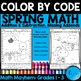May: Math "May"hem ~ Spring Math Printables Color By Codes PuzzlesSubject
Resource Type
Product Rating
File Type

PDF (Acrobat) Document File

6 MB|23 pages
Share
Product Description
May: Math "May"hem: Spring Math Printables ~ Color By The Code Puzzles To Practice Addition and Subtraction Facts

~This Color By Number Unit Is Aligned To The CCSS. Each Page Has The Specific CCSS Listed.~

This set includes 10 math puzzles....Spring, Mother's Day, Cinco de Mayo!

Set also includes 10 answer keys for the 10 puzzles.

Common Core Standards Details

CCSS.Math.Content.1.OA.B.3 Apply properties of operations as strategies to add and subtract. Examples: If 8 + 3 = 11 is known, then 3 + 8 = 11 is also known. (Commutative property of addition.) To add 2 + 6 + 4, the second two numbers can be added to make a ten, so 2 + 6 + 4 = 2 + 10 = 12. (Associative property of addition.)

CCSS.Math.Content.1.OA.C.6 Add and subtract within 20, demonstrating fluency for addition and subtraction within 10. Use strategies such as counting on; making ten (e.g., 8 + 6 = 8 + 2 + 4 = 10 + 4 = 14); decomposing a number leading to a ten (e.g., 13 – 4 = 13 – 3 – 1 = 10 – 1 = 9); using the relationship between addition and subtraction (e.g., knowing that 8 + 4 = 12, one knows 12 – 8 = 4); and creating equivalent but easier or known sums (e.g., adding 6 + 7 by creating the known equivalent 6 + 6 + 1 = 12 + 1 = 13).

CCSS.Math.Content.2.OA.B.2 Fluently add and subtract within 20 using mental strategies. By end of Grade 2, know from memory all sums of two one-digit numbers.

CCSS.Math.Content.2.NBT.B.5 Fluently add and subtract within 100 using strategies based on place value, properties of operations, and/or the relationship between addition and subtraction.

CCSS.Math.Content.3.NBT.A.2 Fluently add and subtract within 1000 using strategies and algorithms based on place value, properties of operations, and/or the relationship between addition and subtraction.

Click on the preview to see the puzzles.

~~~~~~~~~~~~~~~~~~~~~~~~~~~~~~~~~~~~~~~~~~~~~~~~~~~~~~~~~

Are you interested in getting ALL of the “Color-By-The-Code” Math Puzzles in my store?

By popular demand, I have put every one of my math puzzles together for one purchase! This bundle option also gives you lifetime access of every new math puzzle I create with nothing more to buy ever!!

Math Puzzle Palooza: My Entire Store Of "Color-By-The-Code" Math Puzzles!

** If you like color by the code puzzles, but you do not want to buy the bundle, you might be interested in my individual packets which are listed here:

My Complete Color By The Code Math Puzzle List

~~~~~~~~~~~~~~~~~~~~~~~~~~~~~~~~~~~~~~~~~~~~~~~~~~~~~~~~~

~ If you would like to be notified when my products are updated, freebies are offered and new items are added to my store, then follow me! ~

Thank you!

Irene Hines

Teaching Affects Eternity
Total Pages
23 pages